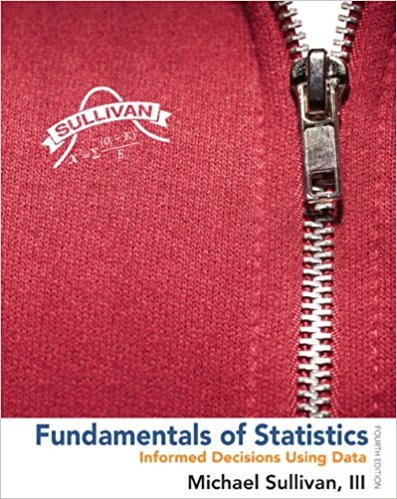×
×

# Solutions for Chapter Chapter 11.3: Fundamentals of Statistics 4th Edition## Full solutions for Fundamentals of Statistics | 4th Edition

ISBN: 9780321838704Solutions for Chapter Chapter 11.3

Solutions for Chapter Chapter 11.3
4 5 0 308 Reviews
26
3
##### ISBN: 9780321838704

Chapter Chapter 11.3 includes 26 full step-by-step solutions. This expansive textbook survival guide covers the following chapters and their solutions. This textbook survival guide was created for the textbook: Fundamentals of Statistics, edition: 4. Since 26 problems in chapter Chapter 11.3 have been answered, more than 310243 students have viewed full step-by-step solutions from this chapter. Fundamentals of Statistics was written by and is associated to the ISBN: 9780321838704.

Key Statistics Terms and definitions covered in this textbook
• Additivity property of x 2

If two independent random variables X1 and X2 are distributed as chi-square with v1 and v2 degrees of freedom, respectively, Y = + X X 1 2 is a chi-square random variable with u = + v v 1 2 degrees of freedom. This generalizes to any number of independent chi-square random variables.

• Analytic study

A study in which a sample from a population is used to make inference to a future population. Stability needs to be assumed. See Enumerative study

• Assignable cause

The portion of the variability in a set of observations that can be traced to speciic causes, such as operators, materials, or equipment. Also called a special cause.

• Biased estimator

Unbiased estimator.

• Conditional mean

The mean of the conditional probability distribution of a random variable.

• Conditional variance.

The variance of the conditional probability distribution of a random variable.

• Conidence interval

If it is possible to write a probability statement of the form PL U ( ) ? ? ? ? = ?1 where L and U are functions of only the sample data and ? is a parameter, then the interval between L and U is called a conidence interval (or a 100 1( )% ? ? conidence interval). The interpretation is that a statement that the parameter ? lies in this interval will be true 100 1( )% ? ? of the times that such a statement is made

• Conidence level

Another term for the conidence coeficient.

• Continuous random variable.

A random variable with an interval (either inite or ininite) of real numbers for its range.

• Control limits

See Control chart.

• Critical region

In hypothesis testing, this is the portion of the sample space of a test statistic that will lead to rejection of the null hypothesis.

• Design matrix

A matrix that provides the tests that are to be conducted in an experiment.

• Error variance

The variance of an error term or component in a model.

• Estimate (or point estimate)

The numerical value of a point estimator.

• Extra sum of squares method

A method used in regression analysis to conduct a hypothesis test for the additional contribution of one or more variables to a model.

• Factorial experiment

A type of experimental design in which every level of one factor is tested in combination with every level of another factor. In general, in a factorial experiment, all possible combinations of factor levels are tested.

• Fraction defective

In statistical quality control, that portion of a number of units or the output of a process that is defective.

• Frequency distribution

An arrangement of the frequencies of observations in a sample or population according to the values that the observations take on

• Generating function

A function that is used to determine properties of the probability distribution of a random variable. See Moment-generating function

• Hat matrix.

In multiple regression, the matrix H XXX X = ( ) ? ? -1 . This a projection matrix that maps the vector of observed response values into a vector of itted values by yˆ = = X X X X y Hy ( ) ? ? ?1 .

×